CBSE Class 12 Sample Paper for 2022 Boards (For Term 2)

Class 12
Solutions of Sample Papers and Past Year Papers - for Class 12 Boards

## Using integration, find the area of the region{(x,y):0≤y≤√3 x, x^2+y^2  ≤4}

This question is similar to Question 34 CBSE Class 12 - Sample Paper 2020 Boards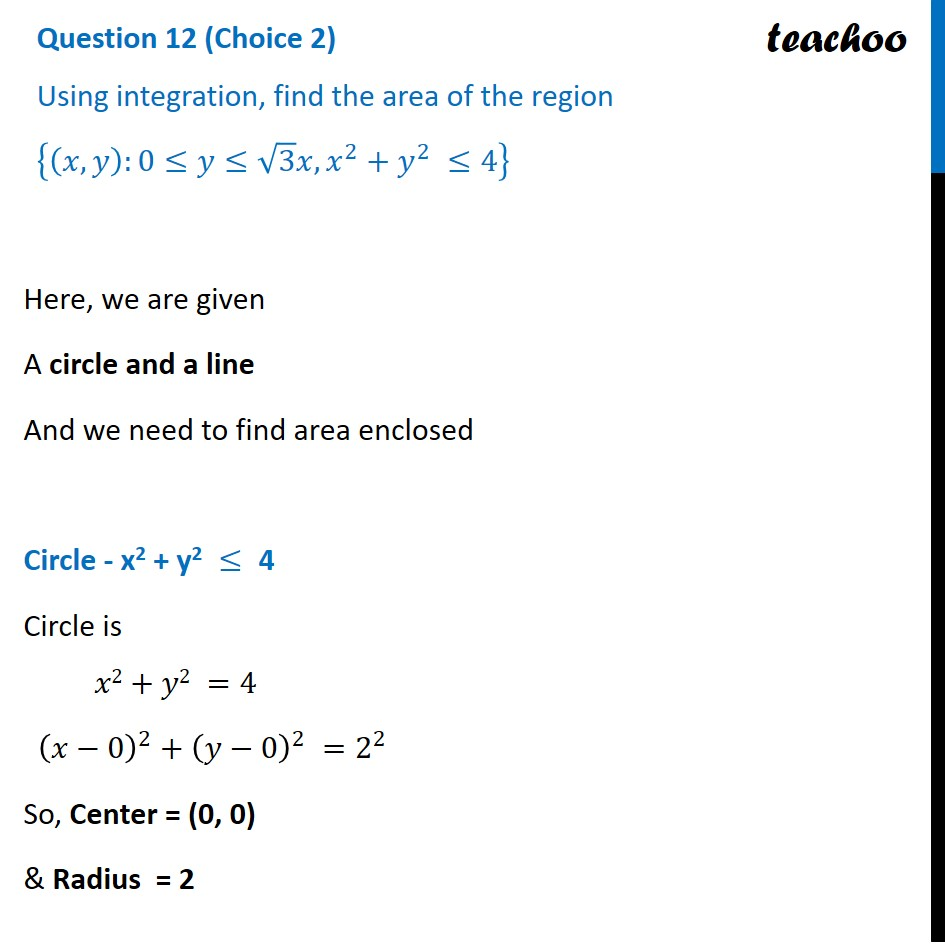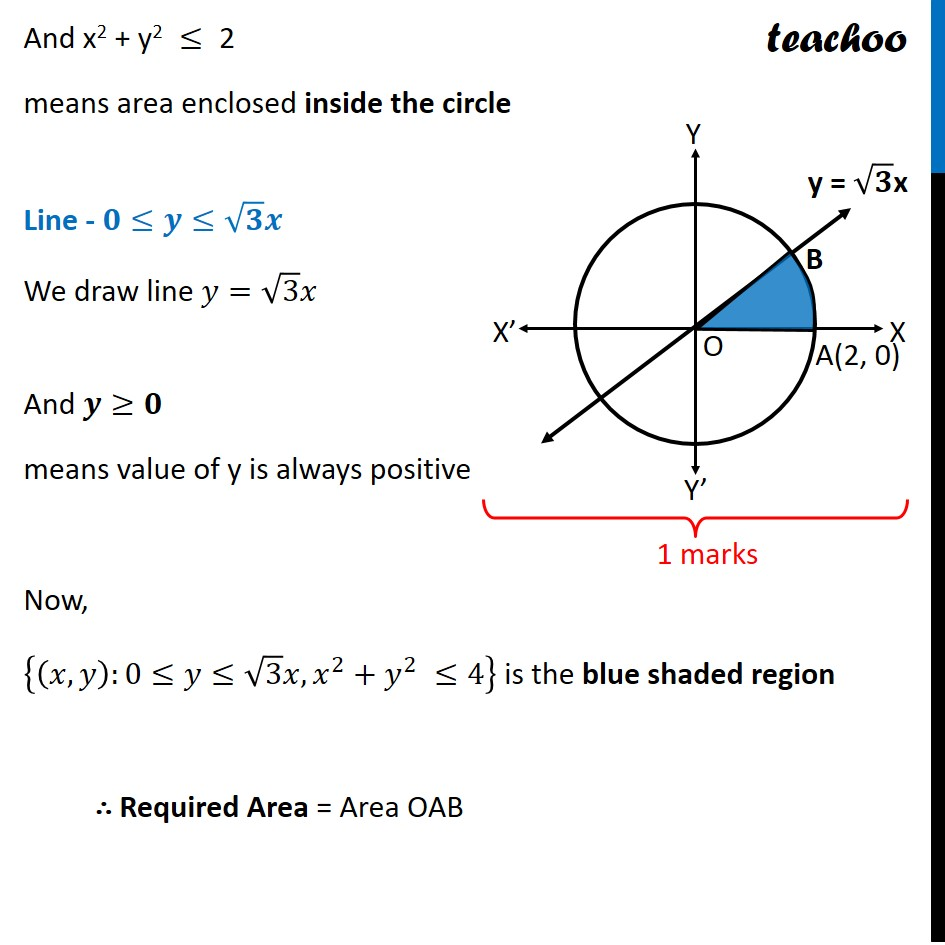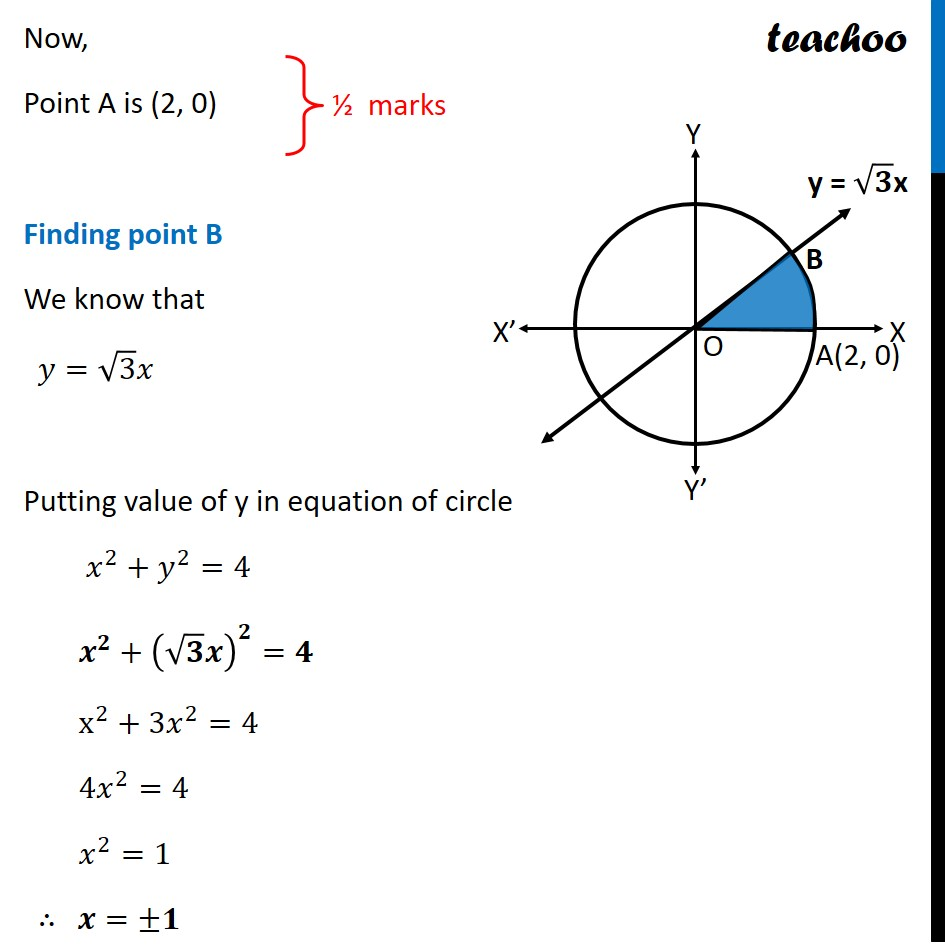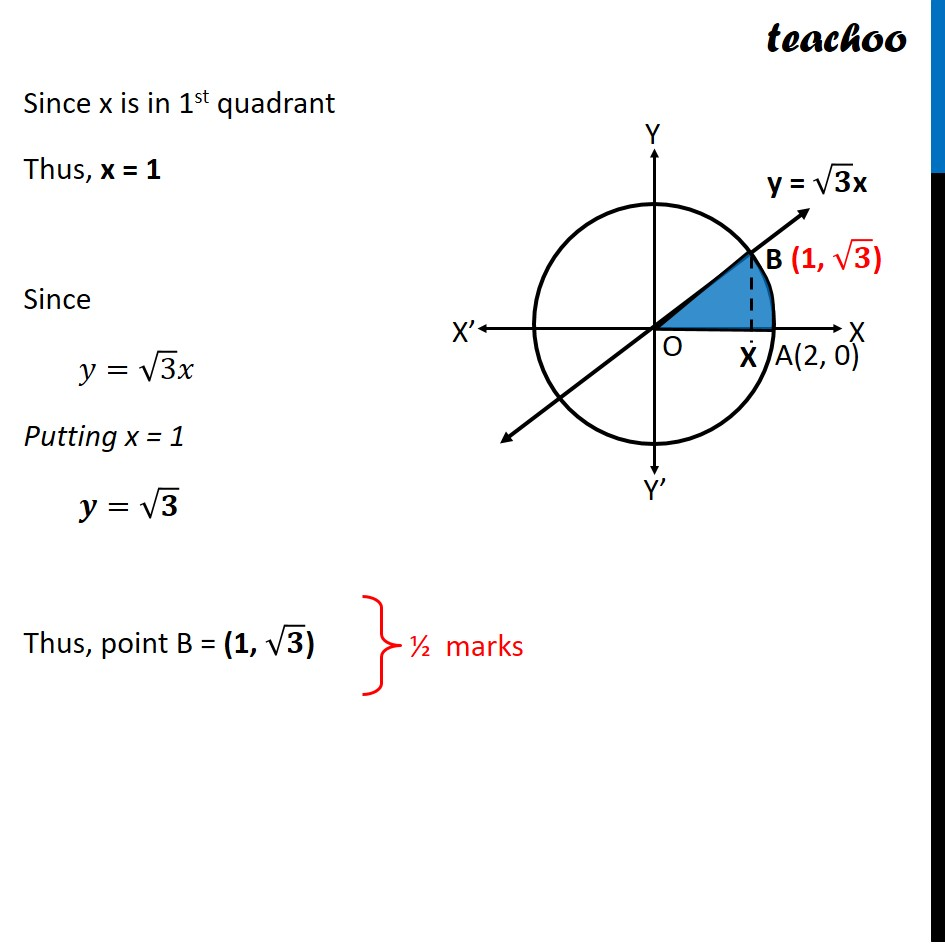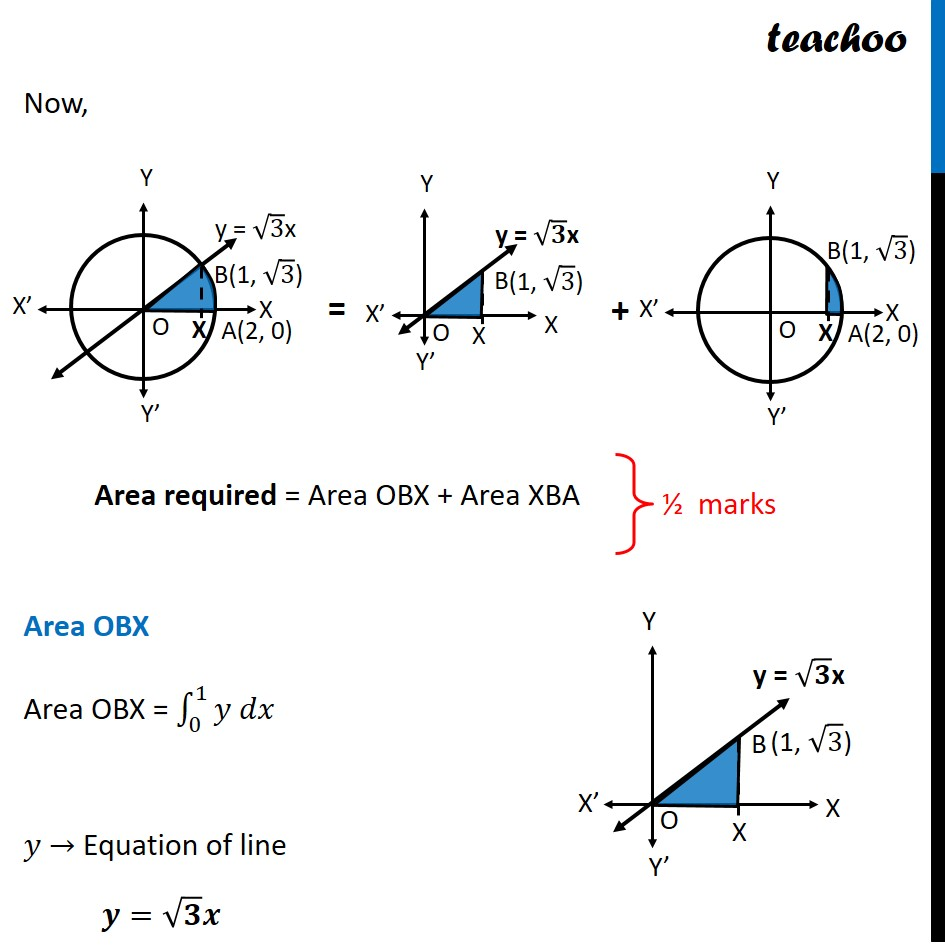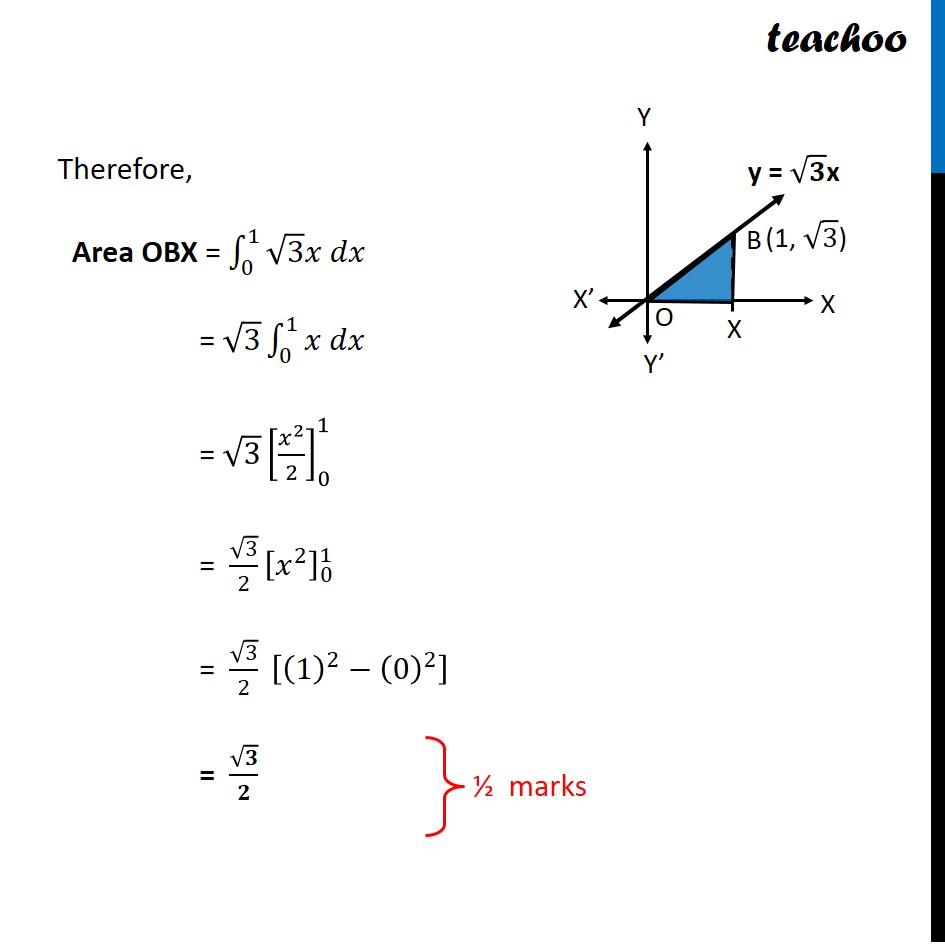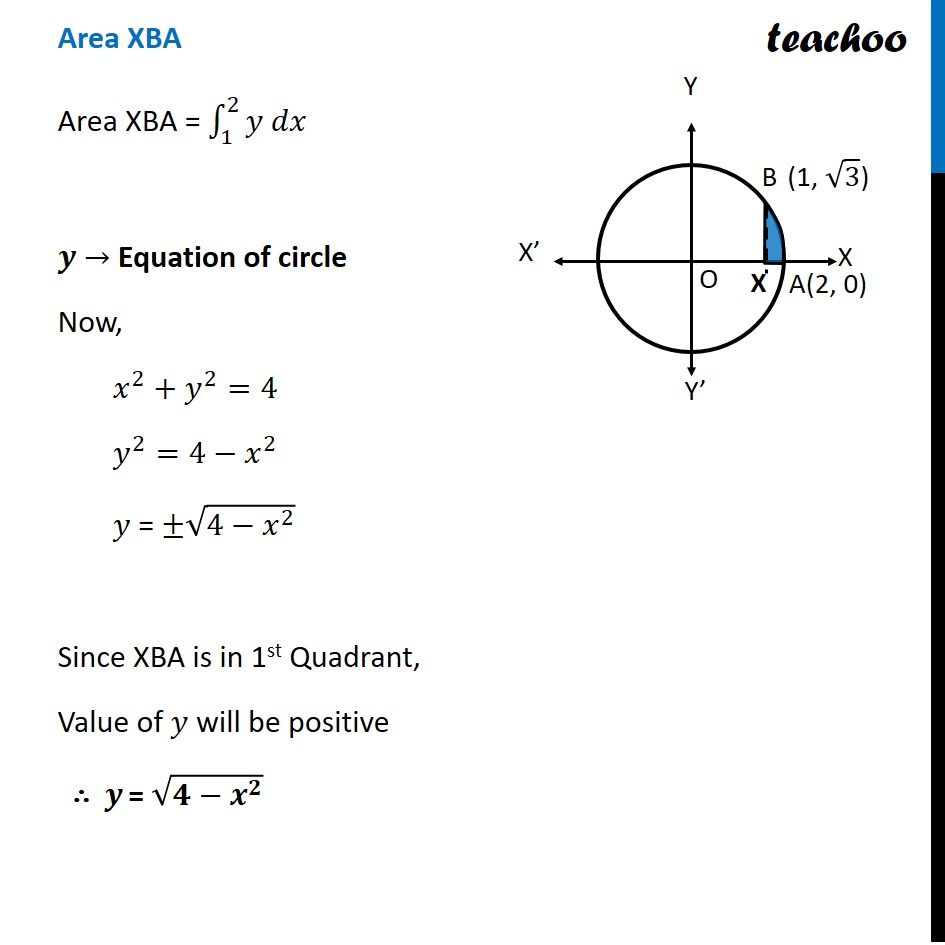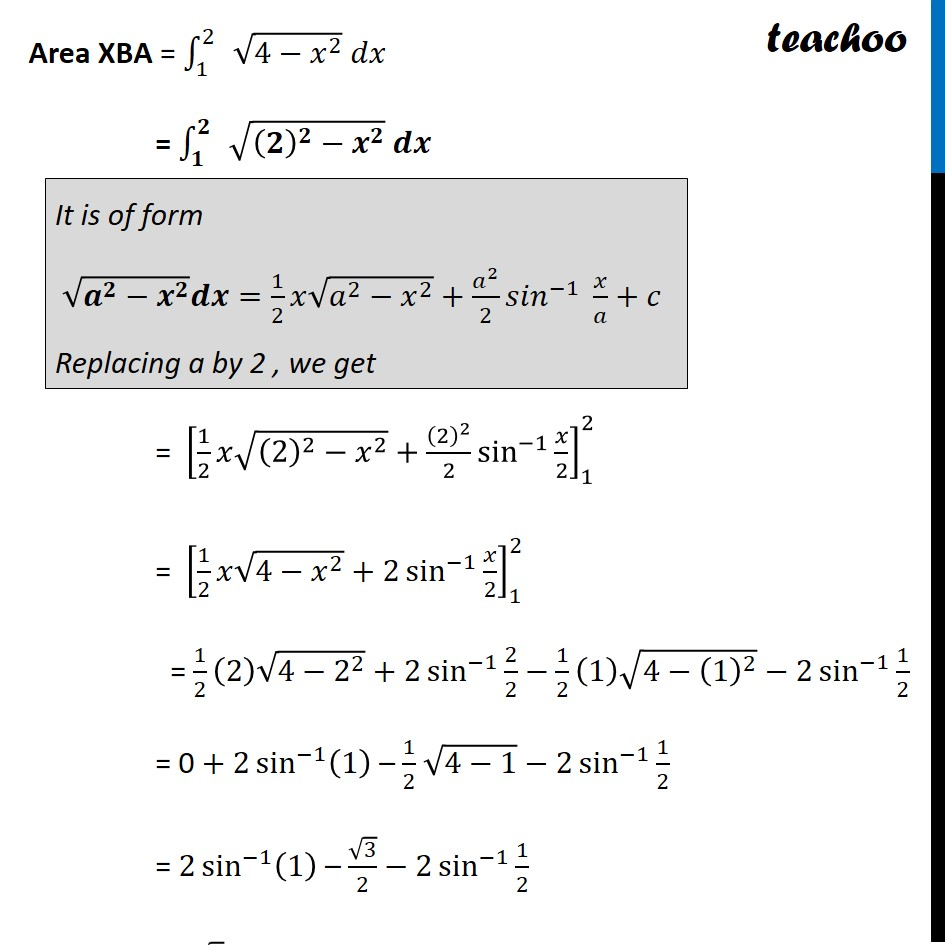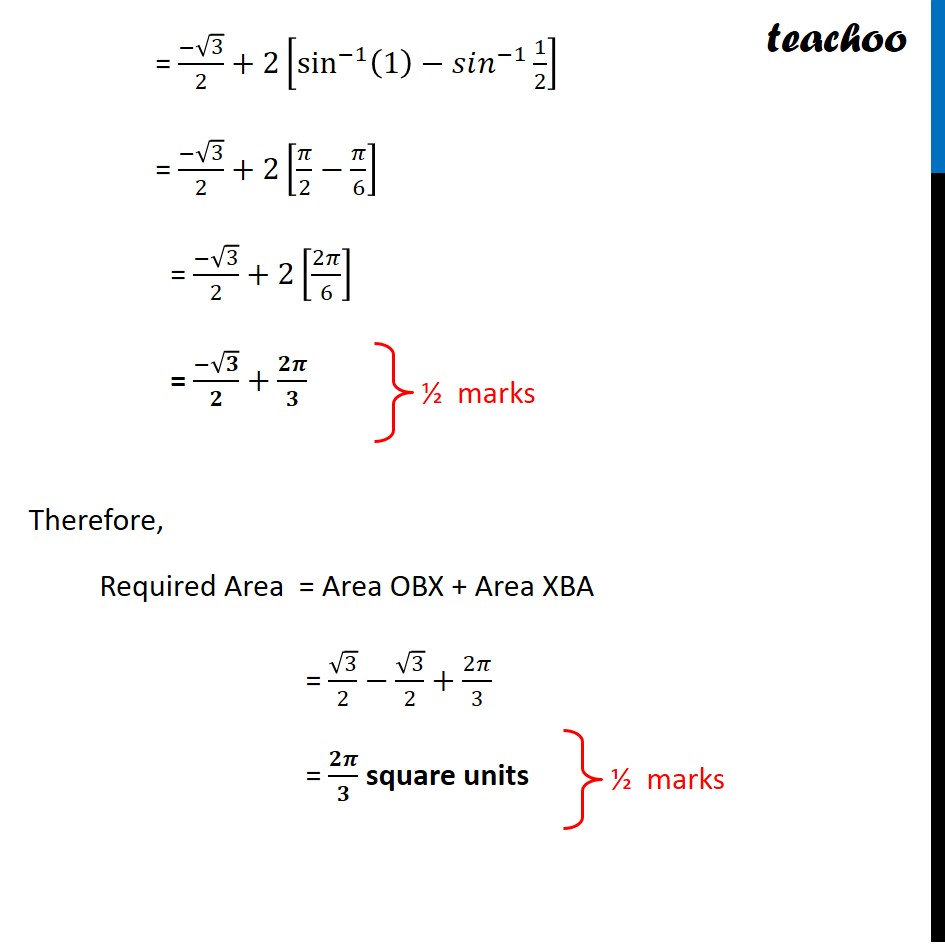Learn in your speed, with individual attention - Teachoo Maths 1-on-1 Class

### Transcript

Question 12 (Choice 2) Using integration, find the area of the region {(𝑥,𝑦):0≤𝑦≤√3 𝑥, 𝑥^2+𝑦^2 ≤4} Here, we are given A circle and a line And we need to find area enclosed Circle - "x2 + y2 "≤" 4" Circle is 𝑥2+𝑦2 =4 (𝑥−0)^2+(𝑦−0)^2 =2^2 So, Center = (0, 0) & Radius = 2 And "x2 + y2 "≤" 2" means area enclosed inside the circle Line - 𝟎≤𝒚≤√𝟑 𝒙 We draw line 𝑦=√3 𝑥 And 𝒚≥𝟎 means value of y is always positive Now, {(𝑥,𝑦):0≤𝑦≤√3 𝑥, 𝑥^2+𝑦^2 ≤4} is the blue shaded region ∴ Required Area = Area OAB Now, Point A is (2, 0) Finding point B We know that 𝑦=√3 𝑥 Putting value of y in equation of circle 𝑥^2+𝑦^2=4 𝒙^𝟐+(√𝟑 𝒙)^𝟐=𝟒 x^2+3𝑥^2=4 4𝑥^2=4 𝑥^2=1 ∴ 𝒙=±𝟏 Since x is in 1st quadrant Thus, x = 1 Since 𝑦=√3 𝑥 Putting x = 1 𝒚=√𝟑 Thus, point B = (1, √𝟑) Now, Area required = Area OBX + Area XBA Area OBX Area OBX = ∫_0^1▒〖𝑦 𝑑𝑥〗 𝑦 → Equation of line 𝒚=√𝟑 𝒙 Therefore, Area OBX = ∫_0^1▒〖√3 𝑥 𝑑𝑥〗 = √3 ∫_0^1▒〖𝑥 𝑑𝑥〗 = √3 [𝑥^2/2]_0^1 = √3/2 [𝑥^2 ]_0^1 = √3/2 [(1)^2−(0)^2 ] = √𝟑/𝟐 Area XBA Area XBA = ∫_1^2▒〖𝑦 𝑑𝑥〗 𝒚 → Equation of circle Now, 𝑥^2+𝑦^2=4 𝑦^2=4−𝑥^2 𝑦 = ±√(4−𝑥^2 ) Since XBA is in 1st Quadrant, Value of 𝑦 will be positive ∴ 𝒚 = √(𝟒−𝒙^𝟐 ) Area XBA = ∫_1^2▒ √(4−𝑥^2 ) 𝑑𝑥 = ∫_𝟏^𝟐▒ √((𝟐)^𝟐−𝒙^𝟐 ) 𝒅𝒙 = [1/2 𝑥√((2)^2−𝑥^2 )+(2)^2/2 sin^(−1)⁡〖𝑥/2〗 ]_1^2 = [1/2 𝑥√(4−𝑥^2 )+2 sin^(−1)⁡〖𝑥/2〗 ]_1^2 = 1/2 (2) √(4−2^2 )+2 sin^(−1)⁡〖2/2〗−1/2 (1) √(4−(1)^2 )−2 sin^(−1)⁡〖1/2〗 = 0 + 2 sin^(−1)⁡(1) – 1/2 √(4−1)−2 sin^(−1)⁡〖1/2〗 = 2 sin^(−1)⁡(1) – √3/2 − 2 sin^(−1)⁡〖1/2〗 = (−√3)/2+2[sin^(−1)⁡〖(1)−𝑠𝑖𝑛^(−1) 〗 1/2]= (−√3)/2+2[sin^(−1)⁡〖(1)−𝑠𝑖𝑛^(−1) 〗 1/2] = (−√3)/2+2[𝜋/2−𝜋/6] = (−√3)/2+2[2𝜋/6] = (−√𝟑)/𝟐+𝟐𝝅/𝟑 Therefore, Required Area = Area OBX + Area XBA = √3/2−√3/2+2𝜋/3 = 𝟐𝝅/𝟑 square units